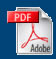Home English Maths Science ICT History Geography Art Year Six Revision Maths APP
Home > Year Six Revision > Year Six Work Page

Welcome to the Year Six Work Page.

This page is for the children to whom I teach mathematics. It is an outline of the work we will be covering over the next half term.

Work for Year Six in the current half term.
Topic Red Group Blue Group Green Group Black Group
Co-ordinates

Co-ordinates in four sectors

Co-ordinates in four sectors with missing vertex co-ordinates in shapes.

Co-ordinates of transformations of shapes

Equations of graphs.Midpoints and Transformations including enlargements.Units

Conversion of metric units

Conversion of metric units

Conversion of metric and Imperial units. Use of conversion graphs.

Conversion of metric, Imperial and currencies using conversion graphs.

Area

Area by counting squares of rectangles and triangles.

Area of rectangles and triangles. Area of irregular shapes.Useful Information for a working wall.

Surface area, volume and edge length of a cuboid (including algebraic). Area of a circle outside a triangle using trig functions.Cellar Problem

Fractions

Recognising fractions. Calculating 1/4, 1/2, 3/4 of a whole number.

Calculating tenths of a whole number.

Adding 1/4, 1/2, 3/4 and whole numbers.

Ordering fractions.

Calculating decimals, fractions and percentages of a rational number.

Ordering Fractions.

Calculating areas of shapes with fractions, decimal fractions.

Fractions investigation.

Ordering Fractions.

Calculate the area of a circle outside a triangle. Look at these in terms of fractions, decimal fractions and percentages.

Cuboid in algebraic terms.

Calculations

Function machines

Revise four number operations.Four number operations of decimal numbers.

Four number operations of fractions, decimal numbers and percentages.

Four number operations of fractions, decimal numbers and percentages.

Time/Geometry

Tellling the time.

Time tables and calendars.

Telling the time.

Time tables and calendars.

Triangles and Circles.

Area and co-ordinates.

Triangles and Circles.

Area and co-ordinates.

Angles and rules involving parallel lines.

Averages and Statistics

Calculate the mode and the median.

Calculate mode, median and mean as well as range.

Calculate mode, median, mean and range.

Draw bar charts and line graphs. Interpret pie charts.

Calculate mode, median, mean and range. Draw pie charts, line graphs and bar charts.

Negative Numbers Negative numbers in context. Ordering negative and positive integers. Four number operations using negative numbers in context. Ordering negative and positive integers and then numbers generally. Four number operations using negative numbers in context. Ordering negative and positive numbers including fractions. Four number operations using negative numbers in context. Ordering negative and positive numbers including fractions.
Data Handling Analyse information using pie charts, bar charts, graphs and sorting diagrams. Analyse information using pie charts, bar charts, graphs and sorting diagrams. Comparing data sets using mode, media, mean. Record collected data using bar charts. Construct a line graph. Analyse information using pie charts, bar charts, graphs and sorting diagrams. Comparing data sets using mode, media, mean. Sort numbers using Venn and Carroll diagrams. Analyse information using pie charts. Draw a pie chart.
Mental Mathematics
Place Value and SI Units Multiplication and division by multiples of 10, 100, 1000 etc. Multiplication and division by multiples of 10, 100, 1000 etc. Conversion of units within decimal system. Multiplication and division by multiples of 10, 100, 1000 etc. Conversion of units within decimal system. Multiplication and division by multiples of 10, 100, 1000 etc. Conversion of units within decimal system.
Shape and Space
Probability and Combinations, Proportion and Ratio